Open in App
Not now

# Three-dimensional Plotting in Python using Matplotlib

• Difficulty Level : Medium
• Last Updated : 15 Mar, 2023

Matplotlib was introduced keeping in mind, only two-dimensional plotting. But at the time when the release of 1.0 occurred, the 3d utilities were developed upon the 2d and thus, we have 3d implementation of data available today! The 3d plots are enabled by importing the mplot3d toolkit. In this article, we will deal with the 3d plots using matplotlib.
Example:

## Python3

 `import` `numpy as np` `import` `matplotlib.pyplot as plt`     `fig ``=` `plt.figure()` `ax ``=` `plt.axes(projection ``=``'3d'``)`

Output: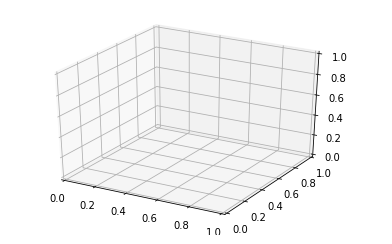With the above syntax three -dimensional axes are enabled and data can be plotted in 3 dimensions. 3 dimension graph gives a dynamic approach and makes data more interactive. Like 2-D graphs, we can use different ways to represent 3-D graph. We can make a scatter plot, contour plot, surface plot, etc. Let’s have a look at different 3-D plots.

## Plotting 3-D Lines and Points

Graph with lines and point are the simplest 3 dimensional graph. ax.plot3d and ax.scatter are the function to plot line and point graph respectively.
Example 1: 3 dimensional line graph

## Python3

 `# importing mplot3d toolkits, numpy and matplotlib` `from` `mpl_toolkits ``import` `mplot3d` `import` `numpy as np` `import` `matplotlib.pyplot as plt`   `fig ``=` `plt.figure()`   `# syntax for 3-D projection` `ax ``=` `plt.axes(projection ``=``'3d'``)`   `# defining all 3 axis` `z ``=` `np.linspace(``0``, ``1``, ``100``)` `x ``=` `z ``*` `np.sin(``25` `*` `z)` `y ``=` `z ``*` `np.cos(``25` `*` `z)`   `# plotting` `ax.plot3D(x, y, z, ``'green'``)` `ax.set_title(``'3D line plot geeks for geeks'``)` `plt.show()`

Output: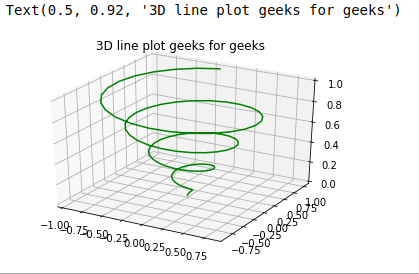Example 2: 3 dimensional scattered graph

## Python3

 `# importing mplot3d toolkits` `from` `mpl_toolkits ``import` `mplot3d` `import` `numpy as np` `import` `matplotlib.pyplot as plt`   `fig ``=` `plt.figure()`   `# syntax for 3-D projection` `ax ``=` `plt.axes(projection ``=``'3d'``)`   `# defining axes` `z ``=` `np.linspace(``0``, ``1``, ``100``)` `x ``=` `z ``*` `np.sin(``25` `*` `z)` `y ``=` `z ``*` `np.cos(``25` `*` `z)` `c ``=` `x ``+` `y` `ax.scatter(x, y, z, c ``=` `c)`   `# syntax for plotting` `ax.set_title(``'3d Scatter plot geeks for geeks'``)` `plt.show()`

Output: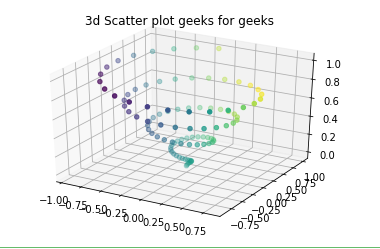## Plotting Surface graphs and Wireframes

Surface graph and Wireframes graph work on gridded data. They take grid value and plot it on three-dimensional surface.
Example 1: Surface graph

## Python3

 `# importing libraries` `from` `mpl_toolkits ``import` `mplot3d` `import` `numpy as np` `import` `matplotlib.pyplot as plt`   `# defining surface and axes` `x ``=` `np.outer(np.linspace(``-``2``, ``2``, ``10``), np.ones(``10``))` `y ``=` `x.copy().T` `z ``=` `np.cos(x ``*``*` `2` `+` `y ``*``*` `3``)`   `fig ``=` `plt.figure()`   `# syntax for 3-D plotting` `ax ``=` `plt.axes(projection ``=``'3d'``)`   `# syntax for plotting` `ax.plot_surface(x, y, z, cmap ``=``'viridis'``, edgecolor ``=``'green'``)` `ax.set_title(``'Surface plot geeks for geeks'``)` `plt.show()`

Output: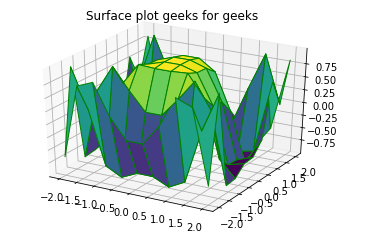Example 2: Wireframes

## Python3

 `from` `mpl_toolkits ``import` `mplot3d` `import` `numpy as np` `import` `matplotlib.pyplot as plt`     `# function for z axis` `def` `f(x, y):` `    ``return` `np.sin(np.sqrt(x ``*``*` `2` `+` `y ``*``*` `2``))`   `# x and y axis` `x ``=` `np.linspace(``-``1``, ``5``, ``10``)` `y ``=` `np.linspace(``-``1``, ``5``, ``10``)` ` `  `X, Y ``=` `np.meshgrid(x, y)` `Z ``=` `f(X, Y)`   `fig ``=` `plt.figure()` `ax ``=` `plt.axes(projection ``=``'3d'``)` `ax.plot_wireframe(X, Y, Z, color ``=``'green'``)` `ax.set_title(``'wireframe geeks for geeks'``);`

Output: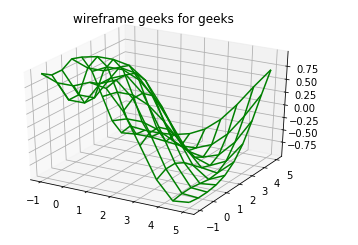## Plotting Contour Graphs

Contour graph takes all the input data in two-dimensional regular grids, and the Z data is evaluated at every point.We use ax.contour3D function to plot a contour graph.
Example:

## Python3

 `from` `mpl_toolkits ``import` `mplot3d` `import` `numpy as np` `import` `matplotlib.pyplot as plt`     `# function for z axis` `def` `f(x, y):` `    ``return` `np.sin(np.sqrt(x ``*``*` `2` `+` `y ``*``*` `3``))`   `# x and y axis` `x ``=` `np.linspace(``-``1``, ``5``, ``10``)` `y ``=` `np.linspace(``-``1``, ``5``, ``10``)` `X, Y ``=` `np.meshgrid(x, y)` `Z ``=` `f(X, Y)`   `fig ``=` `plt.figure()` `ax ``=` `plt.axes(projection ``=``'3d'``)`   `# ax.contour3D is used plot a contour graph` `ax.contour3D(X, Y, Z)`

Output: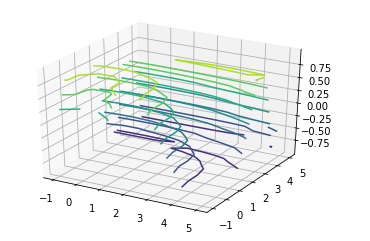## Plotting Surface Triangulations

The above graph is sometimes overly restricted and inconvenient. So by this method, we use a set of random draws. The function ax.plot_trisurf is used to draw this graph. It is not that clear but more flexible.
Example:

## Python3

 `from` `mpl_toolkits ``import` `mplot3d` `import` `numpy as np` `import` `matplotlib.pyplot as plt`     `# angle and radius` `theta ``=` `2` `*` `np.pi ``*` `np.random.random(``100``)` `r ``=` `6` `*` `np.random.random(``100``)`   `# all three axes` `x ``=` `np.ravel(r ``*` `np.sin(theta))` `y ``=` `np.ravel(r ``*` `np.cos(theta))` `z ``=` `f(x, y)`   `ax ``=` `plt.axes(projection ``=``'3d'``)` `ax.scatter(x, y, z, c ``=` `z, cmap ``=``'viridis'``, linewidth ``=` `0.25``);`   `ax ``=` `plt.axes(projection ``=``'3d'``)` `ax.plot_trisurf(x, y, z, cmap ``=``'viridis'``, edgecolor ``=``'green'``);`

Output: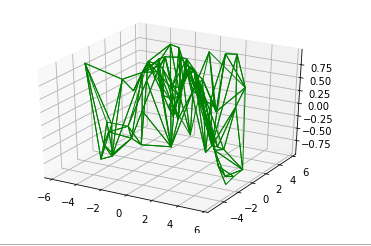## Plotting Möbius strip

Möbius strip also called the twisted cylinder, is a one-sided surface without boundaries. To create the Möbius strip think about its parameterization, it’s a two-dimensional strip, and we need two intrinsic dimensions. Its angle range from 0 to 2 pie around the loop and width ranges from -1 to 1.
Example:

## Python3

 `import` `numpy as np` `import` `matplotlib.pyplot as plt` `from` `mpl_toolkits.mplot3d ``import` `Axes3D`   `# Define the parameters of the Möbius strip` `R ``=` `2`   `# Define the Möbius strip surface` `u ``=` `np.linspace(``0``, ``2``*``np.pi, ``100``)` `v ``=` `np.linspace(``-``1``, ``1``, ``100``)` `u, v ``=` `np.meshgrid(u, v)` `x ``=` `(R ``+` `v``*``np.cos(u``/``2``)) ``*` `np.cos(u)` `y ``=` `(R ``+` `v``*``np.cos(u``/``2``)) ``*` `np.sin(u)` `z ``=` `v ``*` `np.sin(u``/``2``)`   `# Create the plot` `fig ``=` `plt.figure()` `ax ``=` `fig.add_subplot(``111``, projection``=``'3d'``)`   `# Plot the Möbius strip surface` `ax.plot_surface(x, y, z, alpha``=``0.5``)`   `# Set plot properties` `ax.set_xlabel(``'X'``)` `ax.set_ylabel(``'Y'``)` `ax.set_zlabel(``'Z'``)` `ax.set_title(``'Möbius Strip'``)`   `# Set the limits of the plot` `ax.set_xlim([``-``3``, ``3``])` `ax.set_ylim([``-``3``, ``3``])` `ax.set_zlim([``-``3``, ``3``])`   `# Show the plot` `plt.show()`

Output: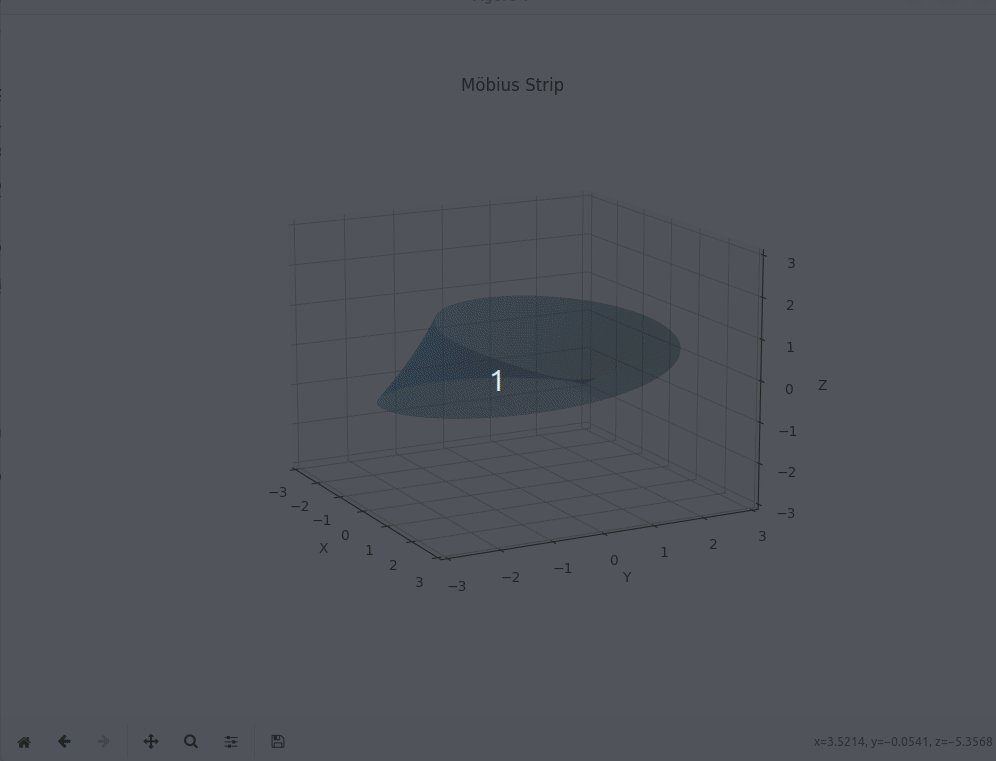My Personal Notes arrow_drop_up
Related Articles